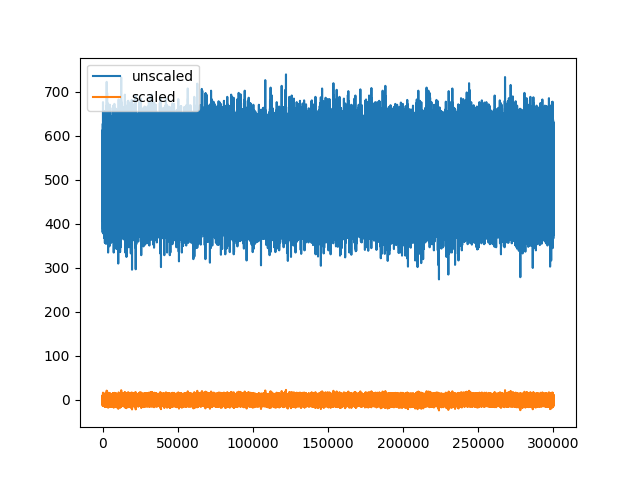# Working with unscaled traces¶

Some file formats store data in convenient types that require offsetting and scaling in order to convert the traces to uV. This example shows how to work with unscaled and scaled traces int `spikeinterface.extractors` module.

```import numpy as np
import matplotlib.pyplot as plt
import spikeinterface.extractors as se
```

First, let’s create some traces in unsigned int16 type. Assuming the ADC output of our recording system has 10 bits, the values will be between 0 and 1024. Let’s assume our signal is centered at 512 and it has a standard deviation of 50 bits

```sampling_frequency = 30000
traces = 512 + 50 * np.random.randn(10 * sampling_frequency, 4)
traces = traces.astype("uint16")
```

Let’s now instantiate a `NumpyRecordingExtractor` with the traces we just created

```recording = se.NumpyRecording([traces], sampling_frequency=sampling_frequency)
print(f"Traces dtype: {recording.get_dtype()}")
```

Out:

```Traces dtype: uint16
```

Since our ADC samples between 0 and 1024, we need to convert to uV. To do so, we need to transform the traces as: traces_uV = traces_raw * gains + offset

Let’s assume that our gain (i.e. the value of each bit) is 0.1, so that our voltage range is between 0 and 1024*0.1. We also need an offset to center the traces around 0. The offset will be: - 2^(10-1) * gain = -512 * gain (where 10 is the number of bits of our ADC)

```gain = 0.1
offset = -2 ** (10 - 1) * gain
```

We are now ready to set gains and offsets to our extractor. We also have to set the `has_unscaled` field to `True`:

```recording.set_channel_gains(gain)
recording.set_channel_offsets(offset)
```

Internally this gains and offsets are handle with properties So the gain could be “by channel”.

```print(recording.get_property('gain_to_uV'))
print(recording.get_property('offset_to_uV'))
```

Out:

```[0.1 0.1 0.1 0.1]
[-51.2 -51.2 -51.2 -51.2]
```

With gains and offset information, we can retrieve traces both in their unscaled (raw) type, and in their scaled type:

```traces_unscaled = recording.get_traces(return_scaled=False)
traces_scaled = recording.get_traces(return_scaled=True)  # return_scaled is True by default

print(f"Traces dtype after scaling: {traces_scaled.dtype}")

plt.plot(traces_unscaled[:, 0], label="unscaled")
plt.plot(traces_scaled[:, 0], label="scaled")
plt.legend()

plt.show()
```Out:

```Traces dtype after scaling: float32
```

Total running time of the script: ( 0 minutes 0.874 seconds)

Gallery generated by Sphinx-Gallery